# 树

• 树是`递归`的，将树的任何一个节点以及节点下的节点都能组合成一个`新的树`。并且很多操作基于递归完成。
• 根节点： 最上面的那个节点(root)，根节点`没有前驱节点`，只有子节点（0个或多个都可以）
• 层数： 一般认为根节点是`第1层`(有的也说第0层)。而树的高度就是层数最高(上图层数开始为1)节点的层数
• 节点关系: `父节点`：就是链接该节点的上一层节点,`孩子节点:`和父节点对应，上下关系。而`祖先节点`是父节点的父节点(或者祖先）节点。`兄弟节点：`拥有同一个父节点的节点们！
• 度： 节点的度就是节点拥有`孩子节点`的个数(是孩子不是子孙).而树的度(最大)节点的度。同时，如果度大于0就成为`分支节点`,度等于0就成为`叶子节点`（没有子孙）。

• 树的节点数=所有节点度数+1.
• 度为m的树第i层最多有m^i-1^个节点。(i>=1)
• 高度而h的m叉树最多（m^h^-1）/(m-1)个节点(`等比数列求和`)
• n个节点的m叉树最小高度[log~m~(n(m-1)+1)]

# 二叉树

• 一：度为2的的树必须有三个节点以上，二叉树可以为空。
• 二：二叉树的度不一定为2：比如说斜树。
• 三：二叉树有左右节点区分，而度为2的树没有左右节点的区分。

• 二叉排序树：树按照一定规则插入排序(本文详解)。
• 平衡二叉树：树上任意节点左子树和右子树深度差距不超过1.

• 非空二叉树叶子节点数=`度为2的节点树+1`.本来一个节点如果度为1.那么一直延续就一个叶子，但如果出现一个度为2除了延续原来的一个节点，会多出一个节点需要维系。所以到`最后会多出一个叶子`
• 非空第i层最多有2^i-1^个节点。
• 高为h的树最多有2^h^-1个节点(等比求和)。
• `完全二叉树`若从左往右，从上到下编号如图：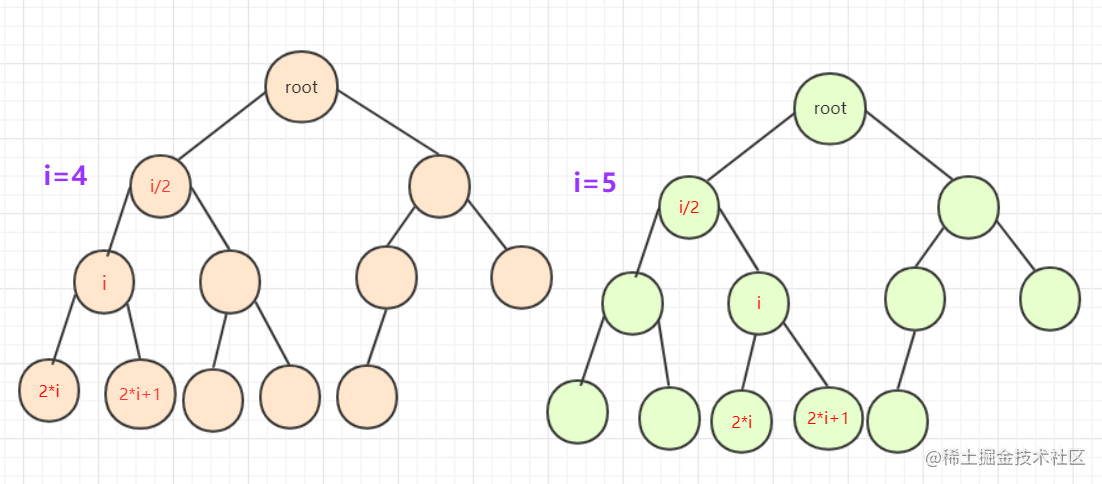# 二叉排序(搜索)树

## 概念

• 从任意节点开始，节点左侧节点值总比节点右侧值要小。 例如。一个二叉排序树依次插入`15，6，23，7，4，71，5，50`会形成下图顺序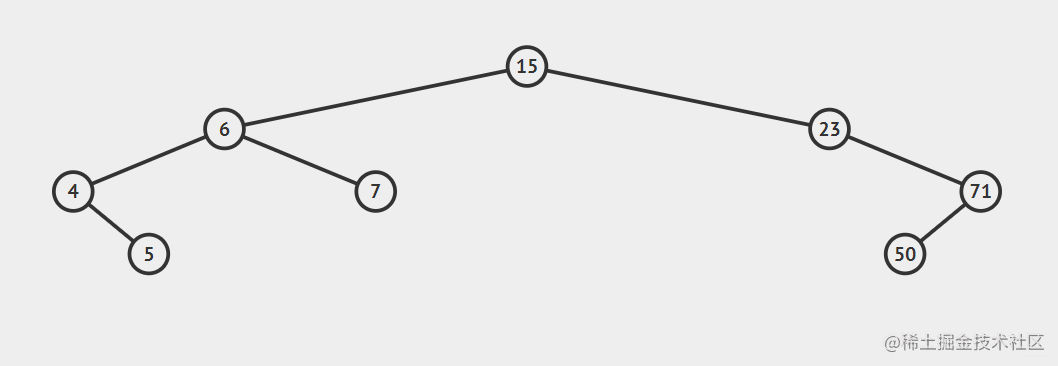## 构造

• 对于node需要这些属性：`left，right，和value`。其中left和right是左右指针，而value是储存的数据，这里用int 类型。

`node`类构造为：

``````class node {//结点
public int value;
public node left;
public node right;
public node()
{
}
public node(int value)
{
this.value=value;
this.left=null;
this.right=null;
}
public node(int value,node l,node r)
{
this.value=value;
this.left=l;
this.right=r;
}
}

``````public class BinarySortTree {
node root;//根
public BinarySortTree()
{root=null;}
public void makeEmpty()//变空
{root=null;}
public boolean isEmpty()//查看是否为空
{return root==null;}
//各种方法
}

## 主要方法

• 既然已经构造号一棵树，那么就需要实现主要的方法。因为二叉排序树中每个节点都能看作一棵树。所以我们创建方法的是时候加上`节点参数`(也就是`函数对每一个节点都能有效`)

### findmax(),findmin()

findmin()找到最小节点：

• 因为所有节点的最小都是往左插入，所以只需要找到最左侧的返回即可。

findmax()找到最大节点：

• 因为所有节点大的都是往右面插入，所以只需要找到最右侧的返回即可。 代码使用递归函数
``````public node findmin(node t)//查找最小返回值是node，调用查看结果时需要.value
{
if(t==null) {return null;}
else if(t.left==null) {return t;}
else return(findmin(t.left));
}
public node findmax(node t)//查找最大
{
if(t==null) {return null;}
else if(t.right==null) {return t;}
else return(findmax(t.right));
}

### isContains(int x)

• 假设我们我们插入x，那么如果存在x我们一定会在查找插入`路径的过程中遇到x`。因为你可以如果已经存在的点，再它的前方会走一次和它相同的步骤。也就是说前面固定，`我来1w次x，那么x都会到达这个位置`。那么我们直接进行查找比较即可！
``````public boolean isContains(int x)//是否存在
{
node current=root;
if(root==null) {return false;}
while(current.value!=x&&current!=null)
{
if(x<current.value) {current=current.left;}
if(x>current.value) {current=current.right;}
if(current==null) {return false;}//在里面判断如果超直接返回
}
//如果在这个位置判断是否为空会导致current.value不存在报错
if(current.value==x) {return true;}
return false;
}

### insert(int x)

``````public node insert(int x)// 插入 t是root的引用
{
node current = root;
if (root == null) {
root = new node(x);
return root;
}
while (current != null) {
if (x < current.value) {
if (current.left == null) {
return current.left = new node(x);}
else current = current.left;}
else if (x > current.value) {
if (current.right == null) {
return current.right = new node(x);}
else current = current.right;
}
}
return current;//其中用不到
}

• 比如说上面结构`插入51`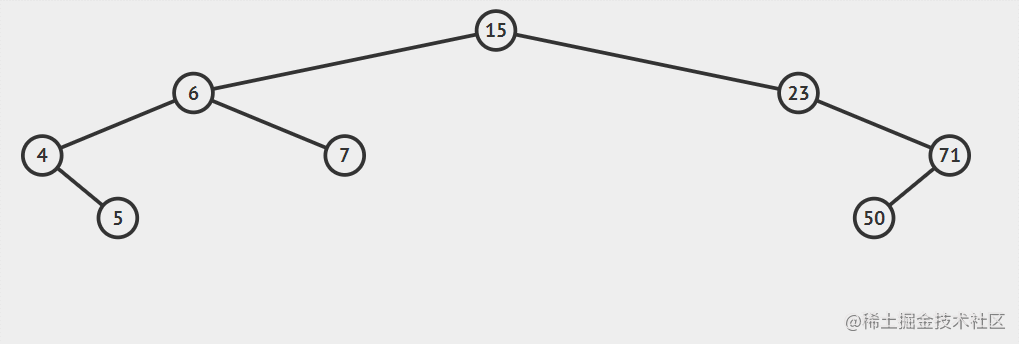### delete(int x)

• 先找到这个点。这个点用这个点的子树可以补上的点填充该点，然后在以这个点为头删除替代的子节点（调用递归）然后在添加到最后情况（只有一个分支，等等）。
• 首先要找到移除的位置，然后移除的那个点`分类讨论`，如果有两个儿子，就选`右边儿子的最左侧那个点替代`，然后再`子树删除替代的那个点`。如果是一个节点，判断是左空还是右空，将这个点`指向不空的那个`。不空的那个就替代了这个节点。入股左右都是空，那么他自己变空null就删除了。

• 这种情况不需要考虑，直接删除即可。(途中红色点)。另`节点=null`即可。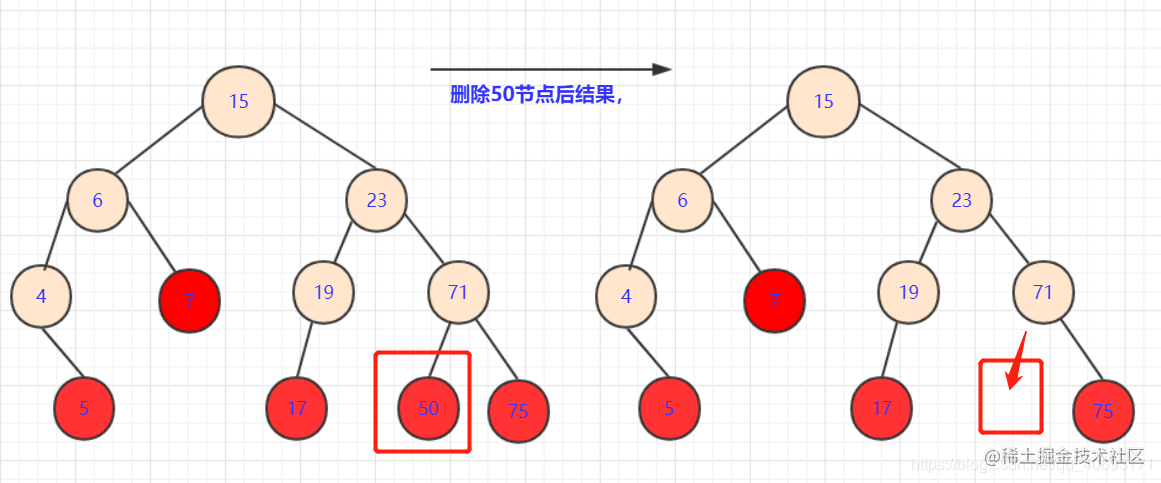• 此种情况也很容易，直接将`删除点的子节点放到被删除位置`即可。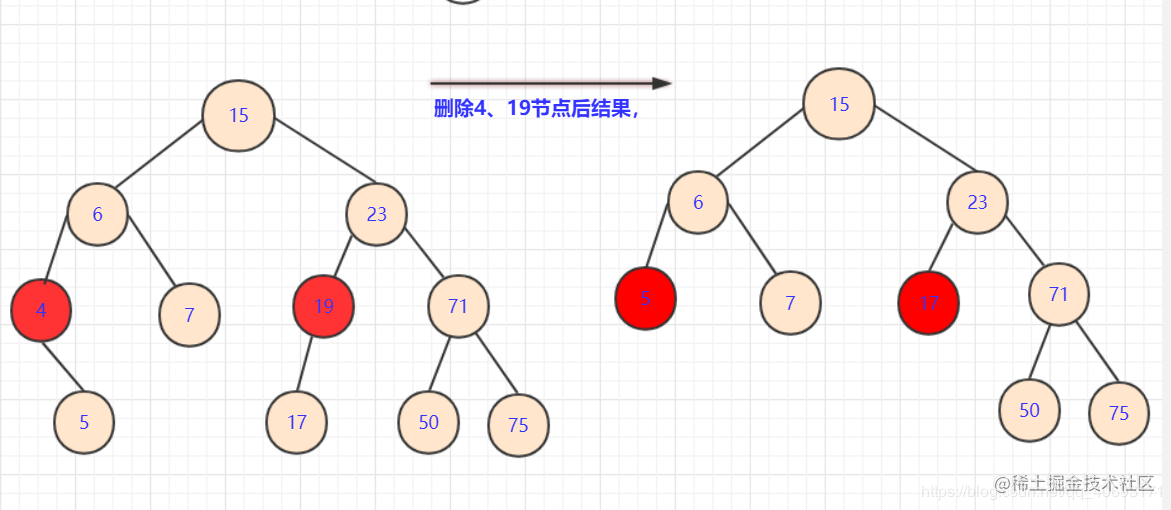• 这种情况相对是复杂的。因为这`涉及`到一个策略问题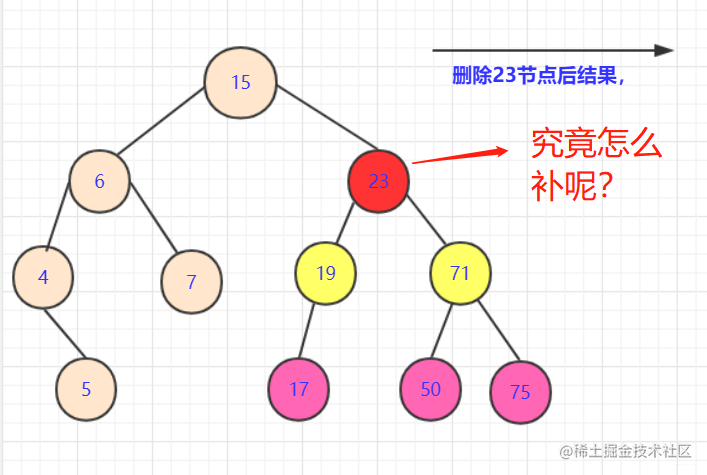• 如果拿`19或者71`节点填补。虽然可以保证部分侧大于小于该节点，但是会引起`合并的混乱`.比如你若用71替代23节点。那么你需要考虑三个节点`(19,50,75)`之间如何处理，还要考虑他们是否满，是否有子女。这是个极其复杂的过程。
• 首先，我们要分析我们要的这个点的属性：能够继承被删除点的所有属性。如果取左侧节点(例如17)那么首先能满足所有右侧节点都比他大（右侧比左侧大）。那么就要再这边`选一个最大的点`让左半枝都比它小。我们分析`左支最大的点`一定是`子树最右侧`
• 如果这个节点是最底层我们很好考虑，可以`直接替换值，然后将最底层的点删除`即可。但是`如果`这个节点`有左枝`。我们该怎么办？
• 这个分析起来也不难，用递归的思想啊。我们删除这个节点，用可以`满足的节点替换`了。会产生什么样的后果？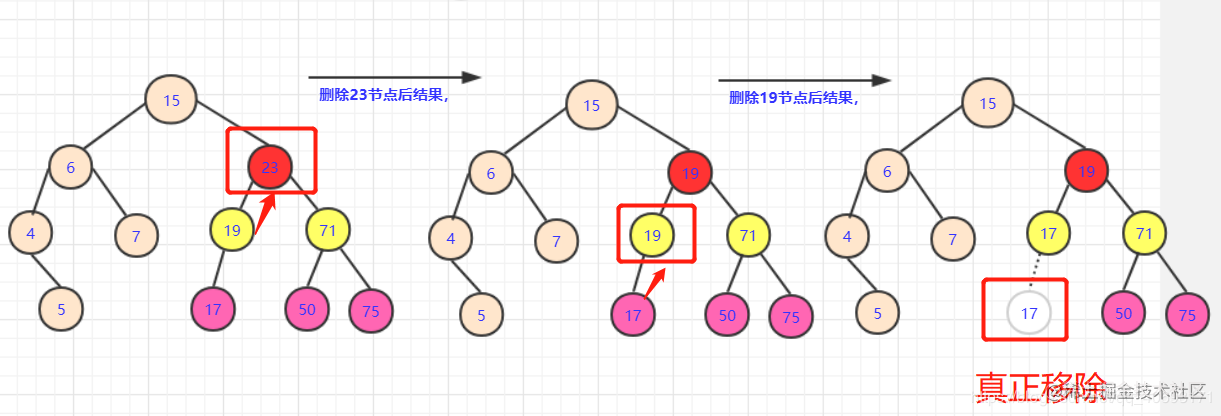• 多出个用过的19节点，转化一下，在左子树中删除`19`的点！那么这个问题又转化为删除节点的问题，查找左子树中有没有能够替代`19`这个点的。

``````public node remove(int x, node t)// 删除节点
{
if (t == null) {
return null;
}
if (x < t.value) {
t.left = remove(x, t.left);
} else if (x > t.value) {
t.right = remove(x, t.right);
} else if (t.left != null && t.right != null)// 左右节点均不空
{
t.value = findmin(t.right).value;// 找到右侧最小值替代
t.right = remove(t.value, t.right);
} else // 左右单空或者左右都空
{
if (t.left == null && t.right == null) {
t = null;
} else if (t.right != null) {
t = t.right;
} else if (t.left != null) {
t = t.left;
}
return t;
}
return t;
}

## 完整代码

``````package 二叉树;

import java.util.ArrayDeque;
import java.util.Queue;
import java.util.Stack;

public class BinarySortTree {
class node {// 结点
public int value;
public node left;
public node right;

public node() {
}

public node(int value) {
this.value = value;
this.left = null;
this.right = null;
}

public node(int value, node l, node r) {
this.value = value;
this.left = l;
this.right = r;
}
}

node root;// 根

public BinarySortTree() {
root = null;
}

public void makeEmpty()// 变空
{
root = null;
}

public boolean isEmpty()// 查看是否为空
{
return root == null;
}

public node findmin(node t)// 查找最小返回值是node，调用查看结果时需要.value
{
if (t == null) {
return null;
} else if (t.left == null) {
return t;
} else
return (findmin(t.left));
}

public node findmax(node t)// 查找最大
{
if (t == null) {
return null;
} else if (t.right == null) {
return t;
} else
return (findmax(t.right));
}

public boolean isContains(int x)// 是否存在
{
node current = root;
if (root == null) {
return false;
}
while (current.value != x && current != null) {
if (x < current.value) {
current = current.left;
}
if (x > current.value) {
current = current.right;
}
if (current == null) {
return false;
} // 在里面判断如果超直接返回
}
// 如果在这个位置判断是否为空会导致current.value不存在报错
if (current.value == x) {
return true;
}
return false;
}

public node insert(int x)// 插入 t是root的引用
{
node current = root;
if (root == null) {
root = new node(x);
return root;
}
while (current != null) {
if (x < current.value) {
if (current.left == null) {
return current.left = new node(x);}
else current = current.left;}
else if (x > current.value) {
if (current.right == null) {
return current.right = new node(x);}
else current = current.right;
}
}
return current;//其中用不到
}

public node remove(int x, node t)// 删除节点
{
if (t == null) {
return null;
}
if (x < t.value) {
t.left = remove(x, t.left);
} else if (x > t.value) {
t.right = remove(x, t.right);
} else if (t.left != null && t.right != null)// 左右节点均不空
{
t.value = findmin(t.right).value;// 找到右侧最小值替代
t.right = remove(t.value, t.right);
} else // 左右单空或者左右都空
{
if (t.left == null && t.right == null) {
t = null;
} else if (t.right != null) {
t = t.right;
} else if (t.left != null) {
t = t.left;
}
return t;
}
return t;
}
}

# 结语

• 这里我们优先学习了树，二叉树，以及二叉搜素树的基本构造。对于二叉搜素树`插入查找比较容易`理解但是实现的时候要注意函数对参数的引用等等。需要认真考虑。
• 而偏有难度的是二叉树的删除，利用一个递归的思想，要找到特殊情况和普通情况，递归一定程度也是`问题的转化`(转成自己相同问题，作用域减小)需要思考。
• 下面还会介绍二叉搜素树的三序遍历(`递归和非递归`).和层序遍历。需要的朋友请持续关注。另外，笔者数据结构专栏欢迎查房。！
• 如果对`后端、爬虫、数据结构算法`等感性趣欢迎关注我的个人公众号交流：`bigsai`。回复爬虫数据结构等有精美资料一份。